﻿ Quantization of the Scalar, Electromagnetic and Dirac Fields in Gravity and the Theory of the Gravitational Gauge Field

### Quantization of the Scalar, Electromagnetic and Dirac Fields in Gravity and the Theory of the Gravit...

M. Khoshsima

International Journal of Physics

## Quantization of the Scalar, Electromagnetic and Dirac Fields in Gravity and the Theory of the Gravitational Gauge Field

Department of Physics, Adelphi University, New York, USA

### Abstract

Real scalar field, EM field and Dirac field can be quantized in gravity. The equation of motion in each field will contain a “gravitational” covariant derivative that will produce new energy-momentum dispersion relation. The dispersion relation in each case preserves the old energy accompanied with a perturbed term. The mathematics of the field quantization will remain unchanged with additional energy perturbation due to interaction with spacetime curvature. The correction of Dirac Lagrangian due to gravitational covariant derivative will lead to the probable existence of the gravitational gauge field, an analog to the EM gauge field.

• M. Khoshsima. Quantization of the Scalar, Electromagnetic and Dirac Fields in Gravity and the Theory of the Gravitational Gauge Field. International Journal of Physics. Vol. 4, No. 5, 2016, pp 135-139. http://pubs.sciepub.com/ijp/4/5/4
• Khoshsima, M.. "Quantization of the Scalar, Electromagnetic and Dirac Fields in Gravity and the Theory of the Gravitational Gauge Field." International Journal of Physics 4.5 (2016): 135-139.
• Khoshsima, M. (2016). Quantization of the Scalar, Electromagnetic and Dirac Fields in Gravity and the Theory of the Gravitational Gauge Field. International Journal of Physics, 4(5), 135-139.
• Khoshsima, M.. "Quantization of the Scalar, Electromagnetic and Dirac Fields in Gravity and the Theory of the Gravitational Gauge Field." International Journal of Physics 4, no. 5 (2016): 135-139.

 Import into BibTeX Import into EndNote Import into RefMan Import into RefWorks

### 1. Introduction

In quantum field theory scalar field, EM field and Dirac field are successfully quantized. However, such quantization neglects gravity. In order to include the effect of gravity in quantization one is required to express Lagrangian and equation of motion (EOM) in (gravitational) covariant form. Consequently, equation of motion will include an additional perturbation term that is due to particle field experiencing spacetime curvature (gravity). One can show that the gravitational covariant form of EOM will lead to the change in energy-momentum dispersion of the particle field, although the mathematics of quantization will remain unchanged.

As we shall see, Lagrangian for real scalar field and EM field remain invariant under gravitational covariant derivative however Dirac Lagrangian will have an addition term that can be corrected by introducing gravitational gauge field. This is “gravitation symmetry” that will make the Dirac Lagrangian invariant under gravitational covariant derivative requirement. In this endeavor the second degree gravitational tensor behavior will not be an obstacle for interactional gauge theory. We shall find out that the gravitation gauge and EM gauge fields are vector analog of one another.

### 2. Quantization of Real Scalar Field in Gravity

Let us recall the free Lagrangian density for the real scalar field,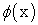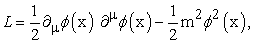(1)

where, m is the mass. Using Euler-Lagrange equation, the equation of motion (EOM) for the above Lagrangian will be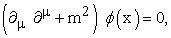(2)

which is known as Klein-Gordon equation. The EOM for a real scalar field in curved spacetime will require the replacement of 4-derivative into “gravitational covariant derivative,”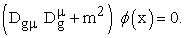(3)

The gravitational covariant derivative in equation (3) can be expanded in the form of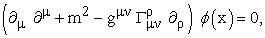(4)

which does preserve the original Lagrangian along with the additional term,being the affine connection . In order to solve Equation (4), we shall express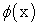in terms of its Fourier transform(5)

Equations (4) and (5) will give us energy-momentum dispersion relation for a real scalar field in gravity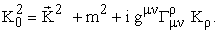(6a)

In the equation above,is the energy of the particle field,is the momentum and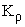is the 4-momentum. Next, we expand the mass in Equation (6a) in terms of 4-momentum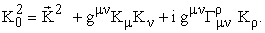(6b)

The last two terms in equation (6b) are tensors and can be combined together into an “effective mass”(7)

Assuming the gravitation contribution manifesting as a perturbation in mass, therefore(8)

Notice that,, whereis the equivalence of gravitational 4-momentum. One can then express the energy of the particle field(9)

We can interpret Equations (8) and (9) such that a scalar field particle in gravity will experience perturbation in its mass and energy. Perturbation being imaginary is perhaps an indication of the decaying nature of particle energy in the gravitational field. Therefore, a scalar field in gravitational field will experience perturbation in mass and energy, with modified energy spectrum of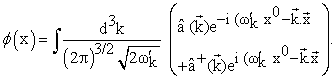(10a)

Consequently, the energy operator will be(10b)

We note that the real scalar field quantization in gravity will remain similar to that of the situation where gravity is absent however the Hamiltonian will change due to perturbation in energy. The particle-gravitational interaction contribution,, can further be simplified by expanding the affine connection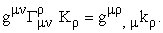(11)

According to Equation (11) the gravitational 4-momentum can now be expressed in terms of derivative of metric tensor,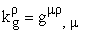. Once we know the metric,the energy perturbation due to gravity can easily be determined.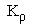, also is the 4-momentum of the field particle. So perturbation in energy in terms of virtual (imaginary) invariant mass is the product of particle field 4-momentum with gravitational field 4-momentum. Now we can express energy-momentum spectrum in terms of the metric(12)

Here, the magnitude of the perturbed energy will be inversely proportional to the magnitude of the unperturbed energy of the scalar field particle(13)

where,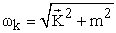.

### 3. Gravitational Symmetry of the Scalar Field

The real scalar field Lagrangian for a free particle (Eqn.(1)) with mass m has a non-continuous symmetry under(14)

Once the interaction term ~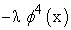is added to the Lagrangian, it is possible to proceed with symmetry breaking. However, we may introduce a new symmetry of different sort regarding the interaction with gravity; “gravitational symmetry.” If Lagrangian remains constant under gravitational covariant requirement, it then will have gravitational symmetry; otherwise physics will require correction by introducing gravitational gauge. Let us take the gravitational covariant derivative form of Equation (1)(15a)

For a scalar field,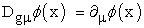. Therefore, real scalar field Lagrangian already has symmetry under gravity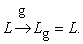(15b)

One can say gravitation gauge correction will not be required for real scalar field Lagrangian. We also will not elaborate on complex scalar field because the physics will remain the same as the real scalar field and therefore redundant.

### 4. Canonical Quantization of the Electromagnetic Field

In order to quantize the EM field in gravity, likewise we need to start with the gravitational covariant of the EM Lagrangian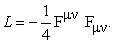(16a)

It is interesting to notice that the gravitational covariant form of the EM tensor will also remain invariant under covariant derivative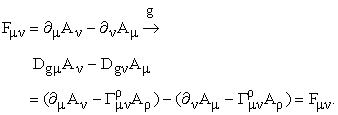(16b)

One can conclude, EM Lagrangian also has symmetry in regards to the gravitational requirement. Once more(16c)

Now, without any further elaboration, we recall the gravitational covariant EOM for EM field(17a)

After expansion of the covariant derivative and simplification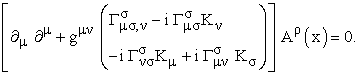(17b)

Once again, the new EOM does preserve the original form with an addition term (in the parenthesis) pertain to gravitational contribution. The energy-momentum spectrum for the above Lagrangian will be(18)

The gravitational contribution in equation (18) can actually be further simplified and expressed in terms metric. As it is expected since we are dealing with a vector filed, the spectrum is gravitationally more complicated, although there is no mass. The perturbation does not manifest in mass-energy, rather, in momentum-energy as should be expect for a photon. The quantization of EM field particle in gravity will remain unchanged although the photon particle field energy will be perturbed by the terms that include affine connection.

### 5. Quantization of Dirac Field

Due to the fact that spinors are not vectors, Dirac field can be more subtle to quantize in gravitational field. Let us express Lagrangian density for a Dirac field in its gravitational covariant derivative form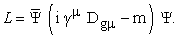(19a)

Notice that the above Lagrangian, unlike real scalar and EM field is not invariant under gravitational covariant derivative as shown below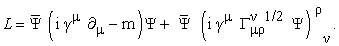(19b)

Instead of spinor in the second term of equation (19b), if we encountered a second degree tensor the analysis would have then been easier. Also note that because there is little “affinity” between tensor and the spinor in the second term of the above equation, we rather change the tensor operation into an invariant scalar, as it will make sense later on. Accordingly one has to take the square root of the affine connection in order to choose proper units (this will also be clear later on)

We have already seen the real scalar field being passive under first gravitational covariant derivative, namely(20a)

On the other hand EM vector field can easily be acted on by a covariant derivative whereas a spinor field is neither scalar nor a vector field and has subtle affinity with tensors. The key is that the Lagrangian has to be a Lorentz scalar otherwise it will not be a valid Lagrangian. In order to resolve this issue we shall contract the last term of Equation (19b) by a (1/1) tensor so that the Lagrangian will remain invariant under Lorentz transformation. Now, not only the Lagrangian is Lorentz invariant, the Dirac field will also be multiplied by scalars only(21)

Again, the half power in the second term is due to unit corrections. In order to proceed with quantization, let us express EOM for Dirac fields in the expanded gravitational covariant form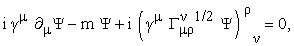(22a)(22b)

As far as perturbation is concerned, Equations (22a) and (22b) are similar. We would concentrate on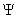only. To make Equation (22a) completely Lorentz invariant we likewise introduce a (1/1) tensor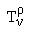in the last term(23)

Similarly, the half power is due to unit correction as it shall be clear later on. As for scalar and EM fields, the above EOM for the Dirac field does contain a perturbed term. One way of being able to analyze this equation is to express the tensorin terms of Dirac field 4-momentum, by analogy to real scalar and EM fields. Let(24)

This is a fair assumption because for real scalar and EM fields it was the 4-momentum of the field that accompanied the affine connection. Simply put, it is the 4-momentums that interact with one another. It happens that for a spinor as compared to a vector, tensor operation is null. After all the perturbation term should include interaction between gravity (affine connection) and the 4-momentum (of the particle field). Naturally we can combine the first two terms in Equation (23)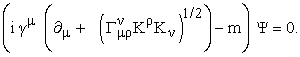(25a)

We now can retrieve modified form of Dirac equation in gravity(25b)

where,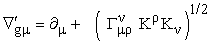. Let us proceed with solving the above equation. We should note that the perturbed component of the equation is due to the Dirac field interacting with gravity. At this point we will express Equations (25) in terms of 4-momentum and we can see why parenthesis in the second term is in half power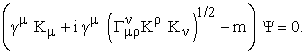(26)

Let us then proceed by expanding the matrices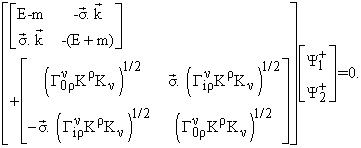(27a)

Where, we have used Dirac matrices in the above equation. Notice that the second matrix is the perturbation due to interaction with gravity. Combining the two matrices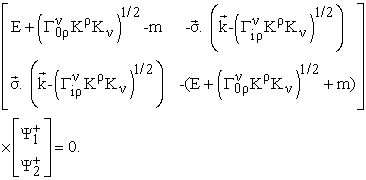(27b)

Equations (27) clearly show the perturbation in spinor energy while experiencing gravitational field. We therefore conclude that the Dirac field quantization in gravity similar to that of scalar and EM fields does remain unchanged, likewise, with having a perturbation in energy spectrum. As a simple example we shall consider a slow moving particle field where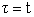, and therefore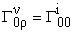. The perturbation term in non-relativistic limit will be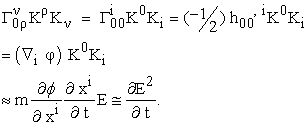(28a)

So the overall perturbation is in the units of classical non-relativistic energy transition. Notice that there can’t be any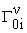component of the affine connection. Inserting the above term in Equation (27b) will give(29)

Equation (29) is the solution to the original Dirac equation plus the perturbation term.

### 6. Gravitational Gauge Field

Let us recall Dirac Lagrangian in gravity, Equation (21)(30a)

In order to correct the perturbation term in the above Lagrangian we shall introduce a new term that will include,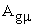the proposed gravitational gauge field with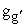being a constant(30b)

Combining the last two terms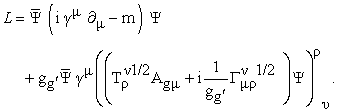(30c)

At this point we also let the gauge transformation(31a)

and multiplying the transformation byfrom the right hand side(31b)

The Lorentz-gravitational invariant Lagrangian then becomes(32a)

The product of the two tensors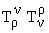is an invariant constant which can be absorbed into,. However, according to Equation (24)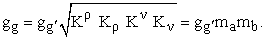(32b)

Where,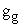, can be defined as the product of strength of the gravitational interaction with the invariant masses of the two Dirac field. Consequently the Lagrangian will become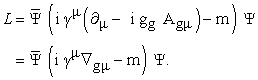(32c)is the proposed gravitational gauge covariant derivative for the above Lagrangian. In order to express the current in the Lagrangian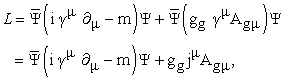(33)

with Dirac currentand the interaction strength,. Finally, gravitation gauge transformation requirement will be as follows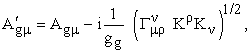(34a)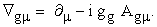(34b)

Equations (34) are analog of local gauge symmetry in QED with EM gauge vector field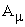. However, here we have introduced gravitational symmetry to generate gravitational gauge vector field. It is also interesting to note that we were successful to generate a gravitational vector field very similar to that of its analog, EM gauge vector field. The key determining factor in this case is the affine connection due to gravity (caused by particles’ mass) interacting with 4-momentum of the particle field. As in electro-dynamics where charge e appears in the interaction so do masses of the two particles in gravitational dynamics between the two particles.

### 7. Conclusion

The quantization of the particle filed in gravity remains unchanged however the energy-momentum dispersion relation of the particle does change. This change or perturbation in energy can be due to interaction of particle filed with the gravitational field. Dirac free particle Lagrangian will require correction due to additional term in Lagrangian caused by external gravity or the gravity of the massive fields. This symmetry of gravity will generate gravitational gauge field which is an analog to that of the EM gauge field.

### References

  M. Schwartz, “Quantum Field Theory and the Standard Model” Cambridge University Press, 4th Printing 2015.In article  S. Hawking, “A Brief History of Time,” New York: Bantam Books, 1988.In article PubMed  R.L. Faber, “Differential Geometry and Relativity Theory,” An Introduction. New York: Marcel Dekker, Inc., 1983.In article  T. Marsh, “General Relativity,” class notes, 2009.In article  “S. Chandrasekhar, “The Mathematical Theory of Black Holes”. Clarendon Press (1983).In article  C.W. Misner, K.S. Thorne, and J.A. Wheeler, “Gravitation”. Freeman (1973).In article  S. Weinberg, “Gravitation and Cosmology: Principles and Applications of the General Theory of Relativity”. Wiley (1972).In article  G. ‘t Hooft, “ Introduction To General Relativity,” Institute for Theoretical Physics; Utrecht University (2002).In article  Poul Olesen, “General Relativity and Cosmology,” The Niels Bohr Institute (2008).In article  M. Kachelrieb, “Gravitation and Cosmology,” Institute for fysikk; NTNU (2010).In article  M. Khoshsima, “Black Hole Spacetime Equation in Special Relativity.” International Journal of Astronomy, Astrophysics and Space Science; Vol. 2, No. 4, 2015, pp. 30-33.In article  M. Khoshsima, “Universe, a Spacetime Harmonic Oscillator.” International Journal of Physics, Vol. 4, no. 1(2016): 21-25.In article